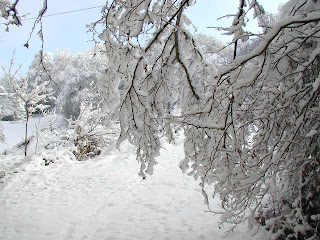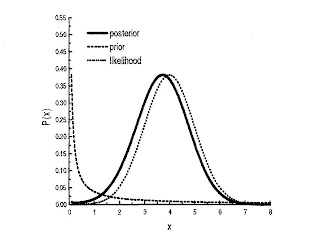## Tuesday, December 22, 2009

### Snow and Bayesian probabilityI was due to deliver a presentation today on the application of Bayesian probability to measurement science. Sadly, that won't now be possible.Still, I'm sure Blogger won't mind me using their resources instead. The basic idea is that there's a distinction between true values x and measured values y.You start off with a prior probability distribution over the true values. You then have a likelihood function, which gives you the probability
P(y|x) of measuring any value y given a hypothetical true value x.When you perform an actual measurement, and obtain a particular measured value y, Bayes's theorem specifies a posterior distribution over the true values. This new distribution can then be set as the prior distribution for the next cycle, and so on. The Bayesian technique is simply a way of representing how knowledge changes and improves in the light of new evidence.In the example represented graphically below, the prior distribution is the steep downward curve, a so-called inverse prior. This shows that prior to performing a measurement, the lowest values have the highest probability. (Technically, you need to truncate this distribution to ensure the total probability is equal to 1).The graph represents the case where you perform an actual measurement, and find that the value is equal to 4 in whatever units are being used. The likelihood function P(y|x) in this example provides a normal distribution over the true values x for a fixed measured value y=4. Bayes's theorem then yields the posterior distribution, which in this case is also basically a normal distribution, but shifted slightly away from 4 towards 0, by the influence of the prior distribution.Sean said...

Ah the Rev. Bayes :0)

I always forget and stick with door 3 (I like the no 3), or is that the monty hall problem?

XiXiDu said...

I assume you are aware of this tutorial:
An Intuitive Explanation of Bayes' Theorem
http://yudkowsky.net/rational/bayes
(Note: Huge page/needs Java)

It's probably the best introduction into Bayes Theorem that exists. Even I could easily follow it and I'm only doing math for less than a year now (still struggling with basic Algebra).

There is a more technical follow-up here:
A Technical Explanation of Technical Explanation
http://yudkowsky.net/rational/technical

Gordon McCabe said...

Excellent, thanks!

XiXiDu said...

I have put a lot more links together here:
http://bit.ly/aIiG4o
Those are the best Bayes resources I know of. There are some really neat visualisations and explanations.

Tried to post this comment twice yesterday evening but blogger.com gave me an error. So if it worked anyway, sorry for flooding.

XiXiDu said...

New one:

'Bayes' Theorem Illustrated (My Way)'
http://lesswrong.com/lw/2b0/bayes_theorem_illustrated_my_way/